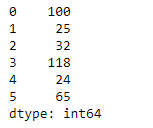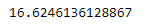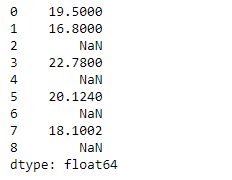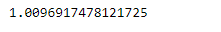# Python | Pandas Series.sem()

Pandas series is a One-dimensional ndarray with axis labels. The labels need not be unique but must be a hashable type. The object supports both integer- and label-based indexing and provides a host of methods for performing operations involving the index.

Pandas` Series.sem()` function return unbiased standard error of the mean over requested axis. The result is normalized by N-1 by default. This can be changed using the ddof argument.

Syntax: Series.sem(axis=None, skipna=None, level=None, ddof=1, numeric_only=None, **kwargs)

Parameter :
axis : {index (0)}
skipna : Exclude NA/null values.
level : If the axis is a MultiIndex (hierarchical), count along a particular level, collapsing into a scalar.
ddof : Delta Degrees of Freedom.
numeric_only : Include only float, int, boolean columns.

Returns : scalar or Series (if level specified)

Example #1 : Use `Series.sem()` function to find the standard error of the mean of the underlying data in the given Series object.

 `# importing pandas as pd ` `import` `pandas as pd ` ` `  `# Creating the Series ` `sr ``=` `pd.Series([``100``, ``25``, ``32``, ``118``, ``24``, ``65``]) ` ` `  `# Print the series ` `print``(sr) `

Output :Now we will use `Series.sem()` function to find the standard error of the mean of the underlying data.

 `# find standard error of the mean ` `sr.sem() `

Output :As we can see in the output, `Series.sem()` function has successfully calculated the standard error the mean of the underlying data in the given Series object.

Example #2 : Use `Series.sem()` function to find the standard error of the mean of the underlying data in the given Series object. The given Series object also contains some missing values.

 `# importing pandas as pd ` `import` `pandas as pd ` ` `  `# Creating the Series ` `sr ``=` `pd.Series([``19.5``, ``16.8``, ``None``, ``22.78``, ``None``, ``20.124``, ``None``, ``18.1002``, ``None``]) ` ` `  `# Print the series ` `print``(sr) `

Output :Now we will use `Series.sem()` function to find the standard error of the mean of the underlying data.

 `# find standard error of the mean ` `# Skip all the missing values ` `sr.sem(skipna ``=` `True``) `

Output :As we can see in the output, `Series.sem()` function has successfully calculated the standard error the mean of the underlying data in the given Series object.

My Personal Notes arrow_drop_upCheck out this Author's contributed articles.

If you like GeeksforGeeks and would like to contribute, you can also write an article using contribute.geeksforgeeks.org or mail your article to contribute@geeksforgeeks.org. See your article appearing on the GeeksforGeeks main page and help other Geeks.

Please Improve this article if you find anything incorrect by clicking on the "Improve Article" button below.